# Summarization

One of the main purposes of data grouping is calculating summaries corresponding to dimension values. For instance, you can sum values, determine the maximum, minimum, average value or count all the values.

To compute these summaries, the Dashboard uses a summary function calculated against the specified measure.

This topic describes how the Dashboard summarizes measure values by default and lists the available summary functions. You will also learn how to change the default summary type.

Note

Note that the Dashboard was not developed to visualize the underlying data, but already grouped / summarized data . If necessary, you can obtain underlying data using a special API of the DashboardViewer or ASPxDashboard controls. To learn more, see the following topics.

## Summarization Overview

For instance, you need to calculate a sum of sales for each dimension value. A sample underlying data source contains the following data.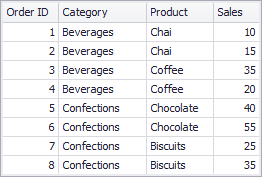If you use the Category field as a dimension and the Sales field as a measure with the Sum summary function, the Dashboard will sum all underlying data source values corresponding to ‘Beverages’ and ‘Confections’.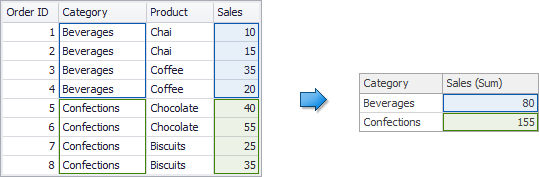If you use the Max summary function, the maximum value corresponding to each category will be selected.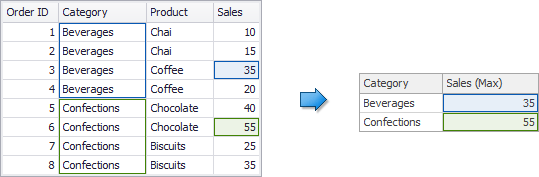## Available Summary Functions

The following summary functions are available.

Note

Values Null and DBNull are excluded from calculations.

 Count The number of values. Count Distinct The number of distinct values. Sum The sum of the values.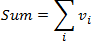Min The smallest value. Max The largest value. Average The average of the values.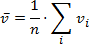StdDev An estimate of the standard deviation of a population where the sample is a subset of the entire population.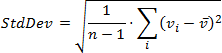StdDevP The standard deviation of a population where the population is the entire data to be summarized.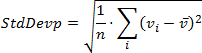Var An estimate of the variance of a population where the sample is a subset of the entire population.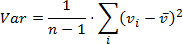VarP The variance of a population where the population is the entire data to be summarized.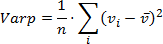Median The median of the values. A median is the number separating the higher half of a value range from the lower half. Mode The mode of the values. A mode is the value that is repeated more often than any other.

## How to Change Summary Type

By default, the Dashboard calculates Sum for numeric measures and Count for measures that contain another type of data. You can change the summary function type in the Dashboard Designer and in code.

## Limitations

Note that changes to the summary type are not available in certain cases.

• You cannot change the summary function type in OLAP mode, because data in a cube is already grouped and summarized.
• Several summary types are not supported for specific data providers in server mode. To learn more, see Data Processing Modes.